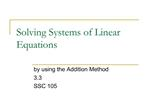Download PresentationSolving Systems of Linear Equations

# Solving Systems of Linear Equations

Download Presentation## Solving Systems of Linear Equations

- - - - - - - - - - - - - - - - - - - - - - - - - - - E N D - - - - - - - - - - - - - - - - - - - - - - - - - - -
##### Presentation Transcript

1. Solving Systems of Linear Equations by using the Addition Method 3.3 SSC 105

2. Solving a System of Equations by the Addition Method Write both equations in standard form Ax + By = C. Clear fractions or decimals (optional). Multiply one or both equations by a number to create opposite coefficients for one of the variables. Add the equations from step 3 to eliminate one variable. Solve for the remaining variable. Substitute the known value found in step 5 into one of the original equations to solve for the other variable. Check the ordered pair in both equations.

3. Example Solve the system by using the addition method. 4x + 5y = 2 3x = 1 � 4y Step 1: Write both equations in standard form. 4x + 5y = 2 3x + 4y = 1 Step 2: There are no fractions or decimals.

4. Example (continued) Solve the system by using the addition method. 4x + 5y = 2 3x = 1 � 4y Step 3: Multiply the first equation by 3. Multiply the second equation by �4. 4x + 5y = 2 12x + 15y = 6 3x + 4y = 1 �12x � 16y = �4 Step 4: Add the equations. 12x + 15y = 6 �12x � 16y = �4 �y = 2